$\newcommand{\Reals}{\mathbb{R}} \newcommand{\Nats}{\mathbb{N}} \newcommand{\PDK}{\mathbf{k}} \newcommand{\IS}{\mathcal{X}} \newcommand{\FM}{\Phi} \newcommand{\Gram}{K} \newcommand{\RKHS}{\mathcal{H}} \newcommand{\prodDot}{\left\langle#1,#2\right\rangle} \DeclareMathOperator*{\argmin}{arg\,min} \DeclareMathOperator*{\argmax}{arg\,max}$

# Motivation: Feature engineering in Machine Learning¶

In ML, one classic way to handle nonlinear relations in data (non-numerical data) with linear methods is to map the data to so called features using a nonlinear function $\FM$ (a function mapping from the data to a vector space).

In :
display(Image(filename="monomials.jpg", width=200))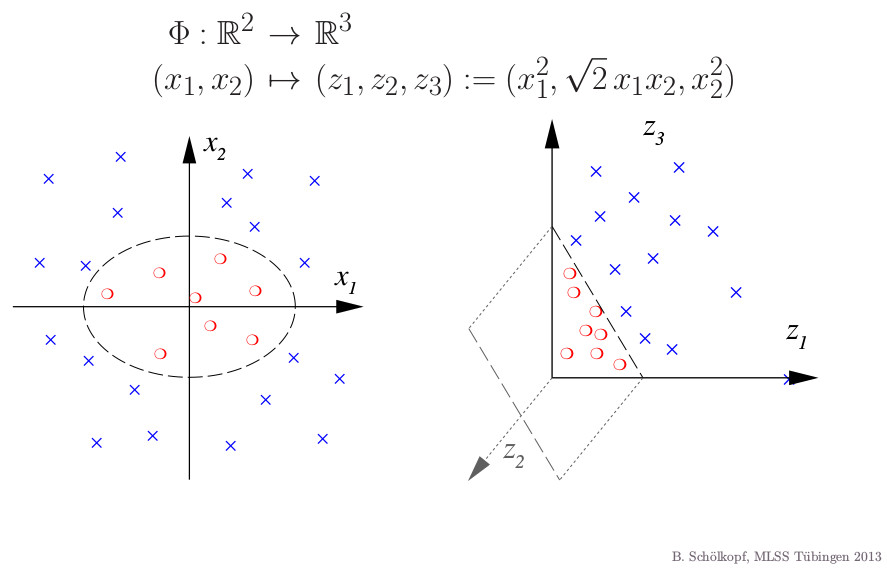In the Feature Space (the domain of $\FM$), we can then use linear algebra, such as angles, norms and inner products, inducing nonlinear operations on the Input Space (codomain of $\FM$). The central thing we need, apart from the feature space being a vector space are inner products, as they induce norms and a possibility to measure angles.

### Simple classification algorithm using only inner products¶

Say we are given data points from the mixture of two distributions with densities $p_0,p_1$: $$x_i \sim w_0 p_0 + w_1 p_1$$ and labels $l_i = 0$ if $x_i$ was actually generated by $p_0$, $l_i = 1$ otherwise. A very simple classification algorithm would be to compute the mean in feature space $\mu_c = \frac{1}{N_c} \sum_{l_i = c} \FM(x_i)$ for $c \in \{0,1\}$ and assign a test point to the class which is the most similar in terms of inner product. In other words, the decision function $f_d:\IS\to\{0,1\}$ is defined by $$f_d(x) = \argmax_{c\in\{0,1\}} \prodDot{\FM(x)}{\mu_c}$$

In :
data = np.vstack([stats.multivariate_normal(np.array([-2,2]), np.eye(2)*1.5).rvs(100),
stats.multivariate_normal(np.ones(2)*2, np.eye(2)*1.5).rvs(100)])
distr_idx = np.r_[*100, *100]

for (idx, c, marker) in [(0,'r', (0,3,0)), (1, "b", "x")]:
pl.scatter(*data[distr_idx==idx,:].T, c=c, alpha=0.4, marker=marker)
pl.arrow(0, 0, *data[distr_idx==idx,:].mean(0), head_width=0.2, head_length=0.2, fc=c, ec=c)
pl.show()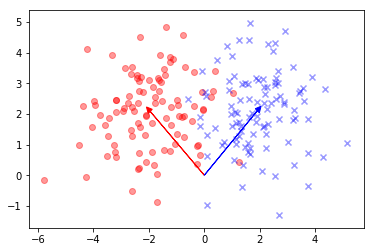Remarkably, all positive definite functions are inner products in some feature space.

Theorem Let $\IS$ be a nonempty set and let $\PDK:\IS\times\IS \to \Reals$, called a kernel. The following two conditions are equivalent:

• $\PDK$ is symmetric and positive semi definite (psd), i.e. for all $x_1, \dots, x_m \in \IS$ the matrix $\Gram$ defined by with entries $\Gram_{i,j} = \PDK(x_i, x_j)$ is symmetric psd $\FM$ is called the Feature Map and $\RKHS_\FM$ the feature space.
• there exists a map $\FM: \IS \to \RKHS_\FM$ to a hilbert space $\RKHS_\FM$ such that $$\PDK(x_i, x_j) = \prodDot{\FM(x_i)}{\FM(x_j)}_\RKHS$$

In other words, $\PDK$ computes the inner product in some $\RKHS_\FM$. We furthermore endow the space with the norm induced by the dot product $\|\cdot\|_\PDK$. From the second condition, it is easy to construct $\PDK$ given $\FM$. A general construction for $\FM$ given $\PDK$ is not as trivial but still elementary.

## Construction of the canonical feature map (Aronszajn map)¶

We give the canonical construction of $\FM$ from $\PDK$, together with a definition of the inner product in the new space. In particular, the feature for each $x \in \IS$ will be a function from $\IS$ to $\Reals$. $$\FM:\IS \to \Reals^\IS\\ \FM(x) = \PDK(\cdot, x)$$ Thus for the linear kernel $\PDK(x,y)=\prodDot{x}{y}$ we have $\FM(x) = \prodDot{\cdot}{x}$ and for the gaussian kernel $\PDK(x,y)=\exp\left(-0.5{\|x-y\|^2}/{\sigma^2}\right)$ we have $\FM(x) = \exp\left(-0.5{\|\cdot -x \|^2}/{\sigma^2}\right)$.

Now $\RKHS$ is the closure of $\FM(\IS)$ wrt. linear combinations of its elements: $$\RKHS = \left\{f: f(\cdot)=\sum_{i=1}^m a_i \PDK(\cdot, x_i) \right\} = span(\FM(\IS))$$ where $m \in \Nats, a_i \in \Reals, x \in \IS$. This makes $\RKHS$ a vector space over $\Reals$.

For $f(\cdot)=\sum_{i=1}^m a_i \PDK(\cdot, x_i)$ and $g(\cdot)=\sum_{i=1}^m' b_j \PDK(\cdot, x'_j)$ we define the inner product in $\RKHS$ as $$\prodDot{f}{g} = \sum_{i=1}^m \sum_{i=1}^m' b_j a_i \PDK(x'_j, x_i)$$ In particular, for $f(\cdot) = \PDK(\cdot,x), g(\cdot) = \PDK(\cdot,x')$, we have $\prodDot{f}{g} = \prodDot{ \PDK(\cdot,x)}{ \PDK(\cdot,x')}=\PDK(x,x')$. This is called the reproducing property of the kernel of this particular $\RKHS$. Obviously $\RKHS$ with this inner product satisfies all conditions for a hilbert space: the inner product is

• positive definite
• linear in its first argument
• symmetric

which is why $\RKHS$ is called a Reproducing Kernel Hilbert Space (RKHS).

### Inner product classification algorithm is equivalent to a classification with KDEs¶

The naive classification algorithm we outlined earlier is actually equivalent to a simple classification algorithm using KDEs. For concreteness, let $\PDK(x,x') = { {{(2\pi )^{-N/2}\left|\Sigma \right|^{-1/2}}}\exp({-{ 0.5}(x-x' )^{\top }\Sigma ^{-1}(x-x' )}})$.

Then the mean in feature space of data from distribution $c$ with the canonical feature map is $$\mu_c = \frac{1}{N_c} \sum_{l_i = c} \FM(x_i) = \frac{1}{N_c} \sum_{l_i = c} \PDK(x_i, \cdot) = \frac{1}{N_c} \sum_{l_i = c} { {{(2\pi )^{-N/2}\left|\Sigma \right|^{-1/2}}}\exp({-{ 0.5}(\cdot-x_i )^{\top }\Sigma ^{-1}(\cdot-x_i )}})$$ which is just a KDE of the density $p_c$ using gaussian kernels with parameter $\Sigma$. For a test point $y$ that we want to classify, its feature is just $\PDK(y,\cdot) = { {{(2\pi )^{-N/2}\left|\Sigma \right|^{-1/2}}}\exp({-{ 0.5}(y-\cdot )^{\top }\Sigma ^{-1}(y-\cdot )}})$. Its inner product with the class mean is just the evaluation of the KDE at $y$ (because of the reproducing property). Thus each point is classified as belonging to the class for which the KDE estimate assigns highest probability to $y$.

In :
class Kernel(object):
def mean_emb(self, samps):
return lambda Y: self.k(samps, Y).sum()/len(samps)

def mean_emb_len(self, samps):
return self.k(samps, samps).sum()/len(samps**2)

def k(self, X, Y):
raise NotImplementedError()

class FeatMapKernel(Kernel):
def __init__(self, feat_map):
self.features = feat_map

def features_mean(self, samps):
return self.features(samps).mean(0)

def mean_emb_len(self, samps):
featue_space_mean = self.features_mean(samps)
return featue_space_mean.dot(featue_space_mean)

def mean_emb(self, samps):
featue_space_mean = self.features(samps).mean(0)
return lambda Y: self.features(Y).dot(featue_space_mean)

def k(self, X, Y):
gram = self.features(X).dot(self.features(Y).T)
return gram

class LinearKernel(FeatMapKernel):
def __init__(self):
FeatMapKernel.__init__(self, lambda x: x)

class GaussianKernel(Kernel):
def __init__(self, sigma):
self.width = sigma

def k(self, X, Y=None):
assert(len(np.shape(X))==2)

# if X=Y, use more efficient pdist call which exploits symmetry
if Y is None:
sq_dists = squareform(pdist(X, 'sqeuclidean'))
else:
assert(len(np.shape(Y))==2)
assert(np.shape(X)==np.shape(Y))
sq_dists = cdist(X, Y, 'sqeuclidean')

K = exp(-0.5 * (sq_dists) / self.width ** 2)
return K

class StudentKernel(Kernel):
def __init__(self, s2, df):
self.dens = dist.mvt(0,s2,df)

def k(self, X,Y=None):
if Y is None:
sq_dists = squareform(pdist(X, 'sqeuclidean'))
else:
assert(len(np.shape(Y))==2)
assert(np.shape(X)==np.shape(Y))
sq_dists = cdist(X, Y, 'sqeuclidean')
dists = np.sqrt(sq_dists)
return exp(self.dens.logpdf(dists.flatten())).reshape(dists.shape)

def kernel_mean_inner_prod_classification(samps1, samps2, kernel):
mean1 = kernel.mean_emb(samps1)
norm_mean1 = kernel.mean_emb_len(samps1)
mean2 = kernel.mean_emb(samps2)
norm_mean2 = kernel.mean_emb_len(samps2)

def sim(test):
return (mean1(test) - mean2(test))

def decision(test):
if sim(test) >= 0:
return 1
else:
return 0

return sim, decision

In :
def apply_to_mg(func, *mg):
#apply a function to points on a meshgrid
x = np.vstack([e.flat for e in mg]).T
return np.array([func(i.reshape((1,2))) for i in x]).reshape(mg.shape)

def plot_with_contour(samps, data_idx, cont_func, method_name, delta = 0.025, pl = pl):
x = np.arange(samps.T.min()-delta, samps.T.max()+delta, delta)
y = np.arange(samps.T.min()-delta, samps.T.max()+delta, delta)
X, Y = np.meshgrid(x, y)
Z = apply_to_mg(cont_func, X,Y)
Z = Z.reshape(X.shape)

# contour labels can be placed manually by providing list of positions
# (in data coordinate). See ginput_manual_clabel.py for interactive
# placement.
fig = pl.figure()
pl.pcolormesh(X, Y, Z > 0, cmap=pl.cm.Pastel2)
pl.contour(X, Y, Z, colors=['k', 'k', 'k'],
linestyles=['--', '-', '--'],
levels=[-.5, 0, .5])
pl.title('Decision for '+method_name)
#plt.clabel(CS, inline=1, fontsize=10)
for (idx, c, marker) in [(0,'r', (0,3,0)), (1, "b", "x")]:
pl.scatter(*data[distr_idx==idx,:].T, c=c, alpha=0.7, marker=marker)

pl.show()

for (kern_name, kern) in [("Linear", LinearKernel()),
("Student-t", StudentKernel(0.1,10)),
("Gauss", GaussianKernel(0.1))
]:
(sim, dec) = kernel_mean_inner_prod_classification(data[distr_idx==1,:], data[distr_idx==0,:], kern)
plot_with_contour(data, distr_idx, sim, 'Inner Product classif. '+kern_name, pl = plt)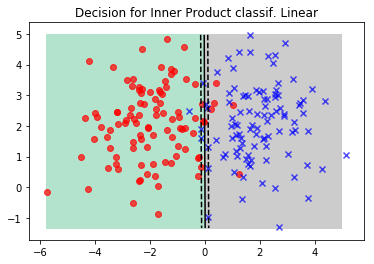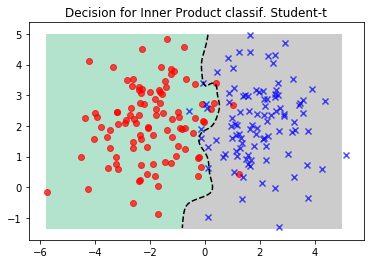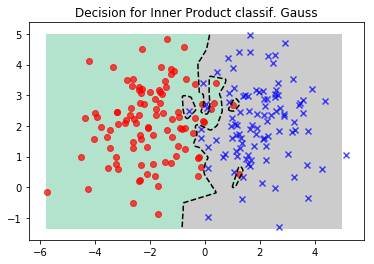Obviously, the linear kernel might be enough already for this simple dataset. Another interesting observation however is that the Student-t based kernel is more robust to outliers of the datasets and yields a lower variance classification algorithm as compared to using a Gaussian kernel. This is to be expected, given the fatter tails of the Student-t. Now lets look at a dataset that is not linearly separable.

In :
data, distr_idx = sklearn.datasets.make_circles(n_samples=400, factor=.3, noise=.05)

for (kern_name, kern) in [("Linear", LinearKernel()),
("Stud", StudentKernel(0.1,10)),
("Gauss1", GaussianKernel(0.1)),
]:
(sim, dec) = kernel_mean_inner_prod_classification(data[distr_idx==1,:], data[distr_idx==0,:], kern)
plot_with_contour(data, distr_idx, sim, 'Inner Product classif. '+kern_name, pl = plt)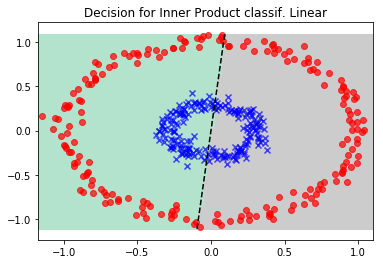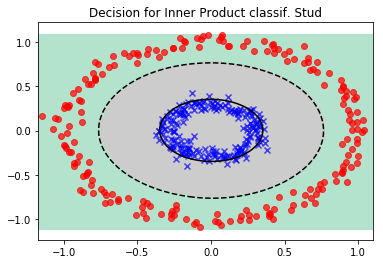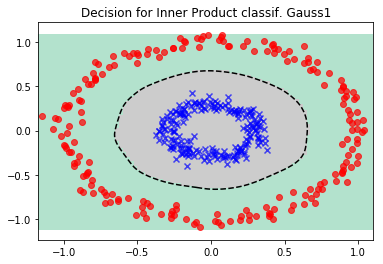In this dataset, the Linear kernel is not a good choice, simply because the classes are not separable linearly in input space. Gaussian and Student-t work, and Student-t shows slightly better robustness properties.

## The kernel mean map¶

One of the objects we looked at, the kernel mean map is particularly interesting. In fact, for so called characteristic kernels, the integral $$\mu_\PDK = \int \PDK(x, \cdot) \mathrm{d}p(x)$$ preserves all information about the distribution $p(x)$, like e.g. characteristic functions, while not even assuming that $x$ is numerical (remember that the only restriction on the codomain of $\PDK$ is that it be nonempty).

When we have an estimator $\widehat{\mu}_\PDK$ and a function $f \in \RKHS$, we have $$\int f(x) \mathrm{d}p(x) \approx \prodDot{f}{\widehat{\mu}_\PDK}$$ because of the reproducing property. In other words, integration for functions in the RKHS $\RKHS$ is just a dot product in $\RKHS$. The unfortunate restriction being that functions in the RKHS are real valued (or complex valued in the more general case).

Lemma If a kernel is strictly positive definite, it is characteristic.

Thus the Gaussian and Student-t kernel and many other kernels induced by densities will be characteristic. The embedding gives us a distance measure between distributions for free.

Definition The Maximum Mean Discrepancy of two distributions given their kernel mean embeddings $\mu_\PDK, \nu_\PDK$ is defined by $$\mathrm{MMD}(\mu_\PDK, \nu_\PDK) = \|\mu_\PDK - \nu_\PDK\|^2_\PDK$$

Furthermore, marginalization, the chain rule and Bayes rule can all be represented as operations in an RKHS. Also, independence tests for random variables operating in a RKHS have been proposed.

In [ ]: